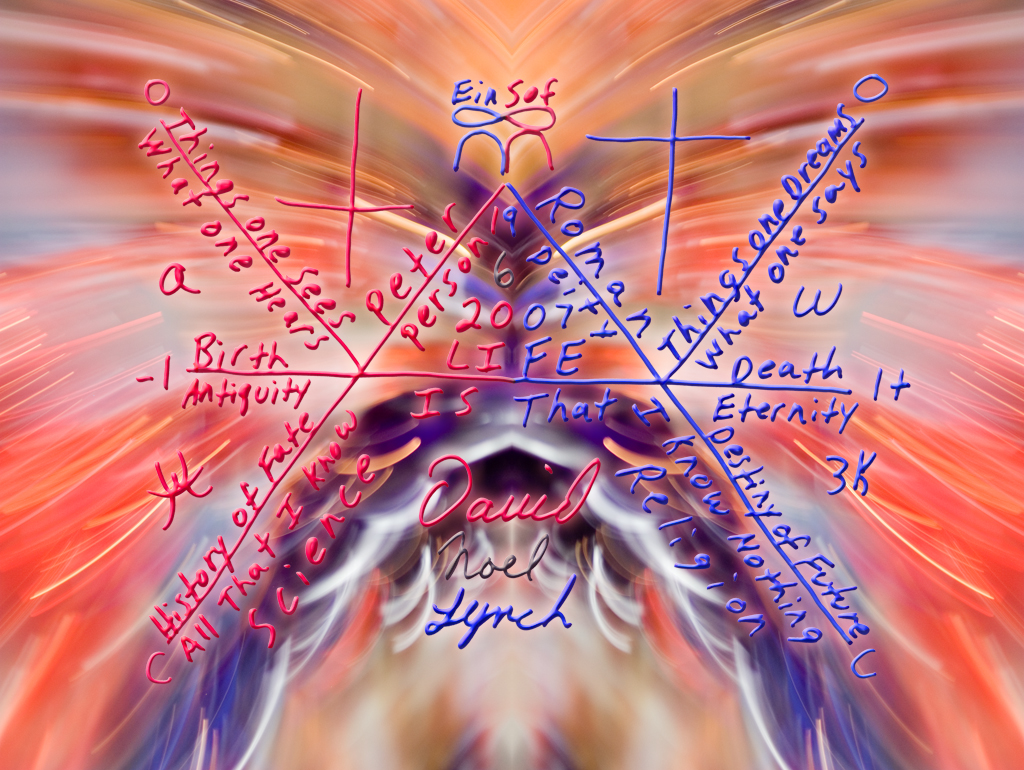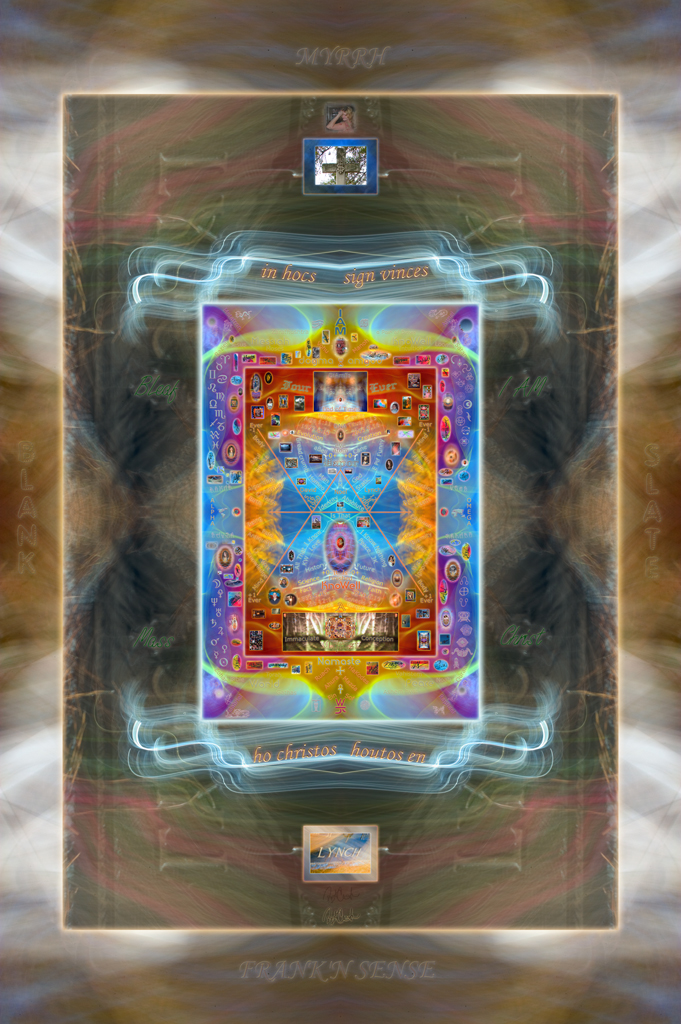Date: Tue, 11 Mar 2008 03:53:08 -0700 (PDT)
Subject: The Quantum Gravity of a Higgs
To: "Edward Witten" <witten at ias.edu>
CC: "Peter Ware Higgs" <P.W.Higgs at ed.ac.uk>
Edward,

While standing on the shoulders of giants, one is likely to get a bloody nose.
Because a large group of people are more than willing to try and knock you off than to give you a good listen.

Over the past few years, I have been working on a diagram for an equation that uses the energy of Einstein,
the force of Newton, and the logic of Socrates to describe a moment of time. I call the equation a KnoWell.http://www.lynchphoto.com/avignon.html

Recently I discovered and Feynman diagram that expresses where the Higgs boson should be found.
If you rotate the graphic at the below link 90 degrees counter clockwise, you may notice a symmetry between it and the KnoWell.
http://en.wikipedia.org/wiki/Image:Gluon-top-higgs.svg

To answer some of the questions revolving around the logic and structure of the KnoWell, I have created a video of me drawing an equation.

What would happen to current models if Einstein would have created an equation for energy that said, ((P(+l+w+h)c)*(F(-l-w-h)c))

Read as, ((Past times mass times the speed of light) times (Future times inverted mass time the speed of light)).
Particle, a, alpha, in the past just under the speed of light, and Wave, w, omega, in the future just over the speed of light.
The only time that E=mc^2 holds true is at the infinite of a moment.

In the KnoWell model, inner space is bubbling out clockwise through quantum loops of gravity,
while outer space is collapsing in counter clockwise through super strings of symmetry.http://www.lynchphoto.com/wreck.html

A graviton would be formed by the super string wave energy from the collapse of outer space as it pushed in to form
a Higgs that was being demanded by a quantum loop of gravity.. Thus is the reason why the stars appear to be red shifted.
Outer space is collapsing.

Flip it around... Gravity is a push from outer space. Magnetism is a pull from inner space.

The KnoWell equation suggests that the universe is in a steady state. Offset by 3 degrees kelvin from being true, absolute, and correct.
This mistake of a universe is warming up with each new Higgs that in turn is expanding space.http://www.lynchphoto.com/gold.html